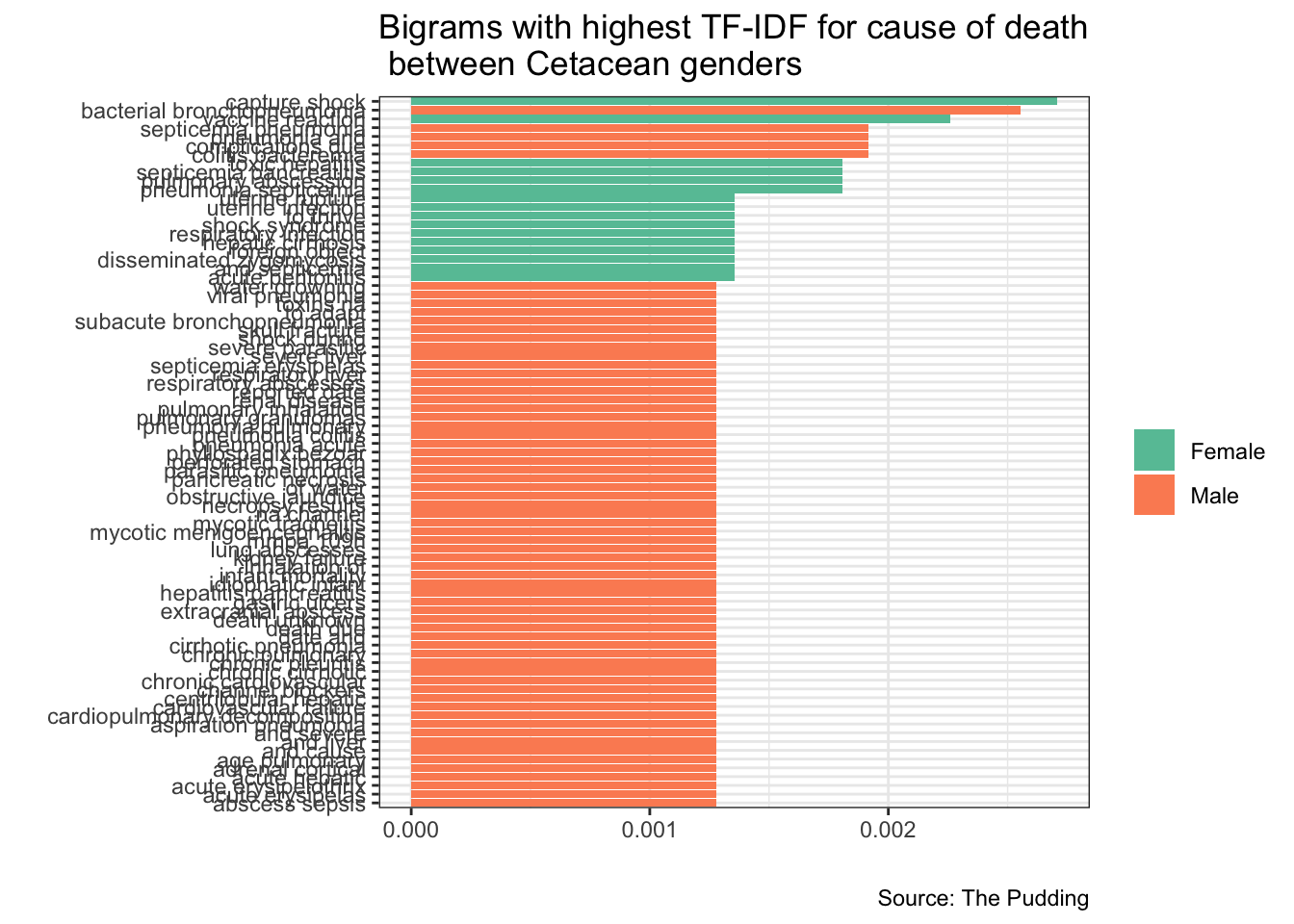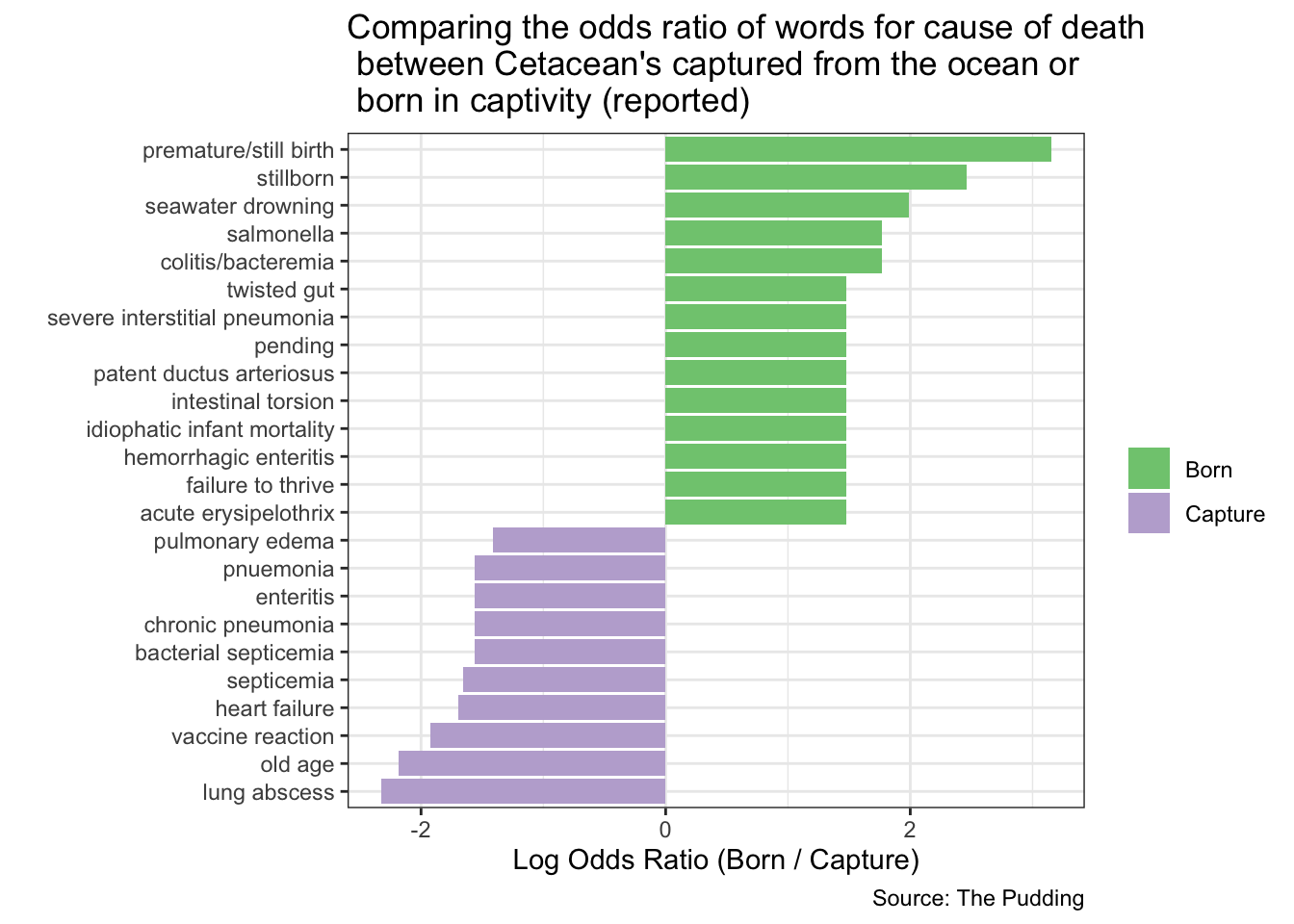Analyzing data for #tidytuesday week of 12/18/2018 (source)

``````# LOAD PACKAGES AND PARSE DATA
library(tidyverse)
library(scales)
library(RColorBrewer)
library(forcats)
library(lubridate)
library(tidytext)

cetaceans <- cetaceans_raw``````

### Most notable cause of death between Male vs Female ?

``````cetaceans %>%
select(sex, COD) %>%
filter(sex != "U") %>%
na.omit() %>%
mutate(sex = replace(sex, str_detect(sex, "F"), "Female"),
sex = replace(sex, str_detect(sex, "M"), "Male")) %>%
unnest_tokens(bigram, COD, token = "ngrams", n = 2) %>%
count(sex, bigram) %>%
bind_tf_idf(bigram, sex, n) %>%
arrange(desc(tf_idf)) %>%
filter(tf_idf > 0.0011) %>%
ggplot() +
geom_col(aes(reorder(bigram, tf_idf), tf_idf, fill = sex)) +
coord_flip() +
scale_fill_brewer(palette = 'Set2',
name = "") +
labs(x = "",
y = "",
title = "Bigrams with highest TF-IDF for cause of death \n between Cetacean genders",
caption = "Source: The Pudding") +
theme_bw() ``````### What is the primary cause of death between Born vs Capture Cetaceans?

``````cod_acquisition_ratio <- cetaceans %>%
select(acquisition, COD) %>%
filter(acquisition == 'Born' | acquisition == 'Capture') %>%
na.omit() %>%
mutate(COD = tolower(COD)) %>%
count(COD, acquisition) %>%
filter(sum(n) >= 10) %>%
ungroup() %>%
spread(acquisition, n, fill = 0) %>%
mutate_if(is.numeric, funs((. + 1) / sum(. +1))) %>%
mutate(logratio = log(Born / Capture)) %>%
arrange(desc(logratio))

cod_acquisition_ratio %>%
arrange(abs(logratio)) %>%
group_by(logratio < 0) %>%
top_n(10, abs(logratio)) %>%
ungroup() %>%
mutate(COD = reorder(COD, logratio)) %>%
ggplot() +
geom_col(aes(COD, logratio, fill = logratio < 0)) +
coord_flip() +
scale_fill_brewer(palette = 'Accent',
name = "",
labels = c("Born", "Capture")) +
theme_bw() +
labs(x = "",
y = "Log Odds Ratio (Born / Capture)",
title = "Comparing the odds ratio of words for cause of death \n between Cetacean's captured from the ocean or \n born in captivity (reported)",
caption = "Source: The Pudding")``````